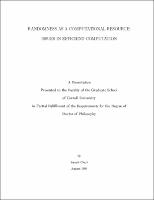## RANDOMNESS AS A COMPUTATIONAL RESOURCE: ISSUES IN EFFICIENT COMPUTATION##### Abstract
Probabilistic methods have become an integral part of theoretical computer science. Typically, the use of randomization leads to solutions which are simple, elegant and more efficient than deterministic algorithms. A new research paradigm in randomized computation is to treat random bits as a resource and design algorithms which use random bits efficiently. The motivations for this approach are that algorithms which use fewer random bits are easier to implement. Also, algorithms using very few random bits can be made deterministic. For several problems all known efficient deterministic algorithms are obtained by this process of derandomization. We design new probabilistic techniques that are used to develop randomness--efficient algorithms for combinatorial optimization problems. The first problem we consider is the abstract problem of isolating one of a possibly exponentially sized set of feasible solutions to the input instance. This tool has been used previously to design algorithms for problems such as perfect matching in graphs, basic problems on matroids and designing random reductions. We design a new technique to solve this problem that is more efficient in the usage of random bits. Using this we derive randomness efficient solutions for the various applications. The randomness complexity of our method is parametrized in terms of the number of feasible solutions. As corollaries we obtain deterministic solutions for special cases of these problems. This new randomness--efficient technique unifies all previously known results for these applications and in many cases gives more efficient algorithms. We prove a strong lower bound which proves that our technique is optimal in the usage of random bits. Another important tool is the concept of random sources whose distribution approximates that of a truly random source. We present a new construction of such sources that is specifically tailored to be easily combined with the algorithmic design method of conditional probabilities. Using this we derive improved deterministic parallel algorithms for several combinatorial optimization problems. We investigate the problem of constructing small sample spaces which satisfy given constraints. For several sets of constraints we present improved constructions of such sample spaces. These have obvious applications to randomness--efficient computation and obtaining deterministic algorithms.
1994-08
##### Publisher
Cornell University
##### Keywords
computer science; technical report
##### Previously Published As
http://techreports.library.cornell.edu:8081/Dienst/UI/1.0/Display/cul.cs/TR94-1449
technical report